IERI Summer Academy 2019 - Sampling

The goals of this Academy are:

1. To provide an introduction to the sampling methodology applied in large-scale educational surveys such as TIMSS, PIRLS, and PISA, its background and significance. The concepts of complex sampling, stratification, and unbiasedness are defined and the selection process is illustrated by means of examples. Further challenges in cross-national comparative studies are highlighted, e.g. the careful definition of target populations and the impact of reduced coverage and participation rates.
2. To demonstrate the necessity of using weights when analyzing data from complex sampling designs. The calculation of sampling weights is explained.
3. To examine the implications for data analysis, particularly the need for specific methods of variance estimation. Jackknife repeated replication (JRR) and balanced repeated replication (BRR) as the most common variance estimation methods applied in large-scale assessments are introduced and participants are pointed to software that can handle these methods.

The topics covered will be the following:

• Sampling design
• Target population definition
• Coverage and exclusions
• Multiple stage cluster sampling
• Systematic sampling
• PPS sampling
• Stratification
• Sample sizes
• Sampling – technical process
• Response rates
• Replacement schools
• Weighting
• Design weights
• Final population weights
• Post-stratification
• Variance estimation
• Introduction
• Variance of the population
• Variance of the sample
• Sampling variance
• Variance estimation in Simple Random Sampling
• Variance estimation in complex samples
• Effect of clusters
• Effect of stratification
• Effect of weights
• Design effect and intraclass correlation coefficient
• Jackknife and Balanced Repeated Replication methods

The general day-to-day schedule will include both presentations and hands-on practical assignments.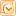Outlook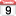iCal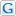Google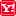Yahoo!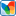MSN
Top